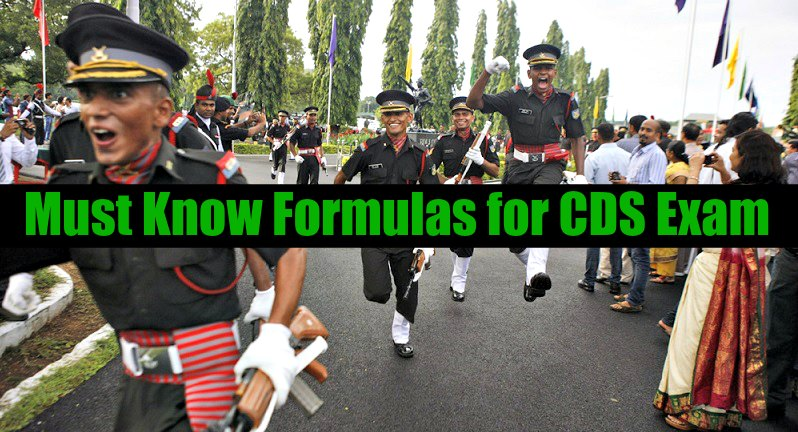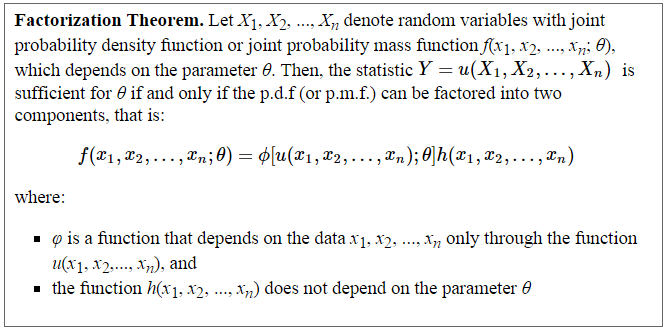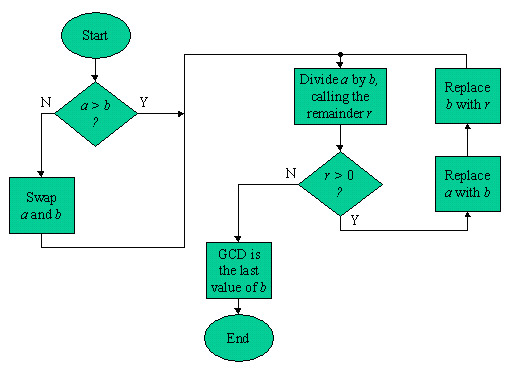# Must Know Formulas for CDS Exam set 1

The UPSC conducts the written exam called CDS Examination for the recruitment of the applicable aspirants in Indian Armed Forces. The exam comprises three basic subjects EnglishGeneral Knowledge and Mathematics. These three subjects include various topics. In this article we will be discussing Must Know Formulas for CDS Exam based on the syllabus.

## CDS Exam Syllabus Details:

Paper 1: English

The objective type question paper is designed to examine the understanding of English of the applicants. English question paper of CDS Exam includes passage for answering few questions related to that passage.

Paper 2: General Knowledge

General Knowledge question paper in CDS examination includes knowledge of current topics and of matters which are observing every day. The aspirants must be able to answer scientific aspects without any particular scientific study. They should have knowledge of History of India and Geographical issues without any specific study. The applicants must be able to answer these general knowledge questions to pass this test.

Paper 3: Elementary Mathematics

The objective type question paper includes various topics of elementary mathematics. Candidates should have posses knowledge about these topics. There are lots of topics to cover which are as following:

• Arithmetic: Aspirants need to perform well in arithmetic which includes Number System, Fundamental Operations, Unitary Methods, and Elementary Number Theorems etc.
• Algebra: The applicants should able to solve basic operations, Remainder Theorem, Quadratic Equations, Linear equations and practical problems etc.
• Geometry: The candidates should able to answer geometric questions which contain Lines and angles, plane and plane figures and their related questions.
• Trigonometry: The applicants need to know the values of Sine x, cosine x and tangent x.
• Mensuration: The all candidates applying for the test should have knowledge of calculating areas of squares, rectangles, triangles, circles and parallelograms etc.
• Statistics: Everyone needs to know the collection and tabulation of data, histograms, pie and bar charts etc.

Must Know Formulas for CDS Exam Set 1 Elementary Number Theory• When a number can be divided up evenly it is a Composite Number
• When a number can not be divided up evenly it is a Prime Number
• A prime number has only two factors: 1 and itself. A composite number has more than two factors. The number 1 is neither prime nor composite.
•  Tests of divisibility by 2, 3, 4, 5, 6, 9 and 11:
1. Divisibility by 2 Rule: Almost everyone is familiar with this rule which states that any even number can be divided by 2. Even numbers are multiples of 2. A number is even if ends in 0,2,4,6, or 8.
2. Divisibility by 3 Rule: A number is divisible by 3 if the sum of its digits is divisible by 3.
3. Divisibility by 4 Rule: A number is divisible by 4 if the number’s last two digits are divisible by 4.
4. Divisibility by 5 Rule: A number is divisible by 5 if its last digit is a 0 or 5.
5. Divisibility by 6 Rule: Since 6 is a multiple of 2 and 3, the rules for divisibility by 6 are a combination of the rule for 2 and the rule for In other words, a number passes this divisibility test only if it passes the test for 2 and test for 3.
6. Divisibility by 9 Rule: A number is divisible by 9 if the sums of the digits are evenly divisible 9.
7. Divisibility by 11 Rule: A number passes the test for 11 if the difference of the sums of alternating digits is divisible by 11.
• Factors are the numbers we can multiply together to get another number.
• A Multiple is the result of multiplying a number by an integer (not a fraction).
• The fundamental theorem of arithmetic, also called the unique factorization theorem or the unique-prime-factorization theorem, states that every integer greater than 1 either is prime itself or is the product of prime numbers, and that this product is unique, up to the order of the factors.• The highest common factor (HCF) of two whole numbers is the largest whole number which is a factor of both.
• The lowest common multiple (LCM) of two whole numbers is the smallest whole number which is a multiple of both.
• Euclid’s algorithm, is an efficient method for computing the greatest common divisor (GCD) of two numbers, the largest number that divides both of them without leaving a remainder. Flow Chart of the algorithm:-• laws of logarithms: There are a number of rules known as the laws of logarithms. These allow expressions involving logarithms to be rewritten in a variety of different ways. The laws apply to logarithms of any base but the same base must be used throughout a calculation.
1. Logb MN = Logb M + Logb N
2. Logb M/N = Logb M – Logb N
3. Logb M = Logb N if and only if M = N
4. Logb Mk = k Logb M
5. Logb b = 1
6. Logb 1 = 0
7. Logb bk = k
8. bLogb x = x

In coming days we will be publishing other sets of CDS syllabus based formulas and important facts under the topic “Must Know Formulas for CDS Exam”, so we request our readers to keep themselves active on our website.#### Ashutosh

Defence exams mentor, cracked CDS and AFCAT exams.

View all Posts